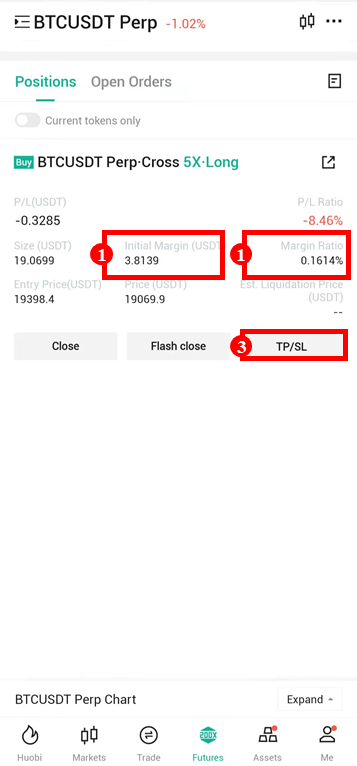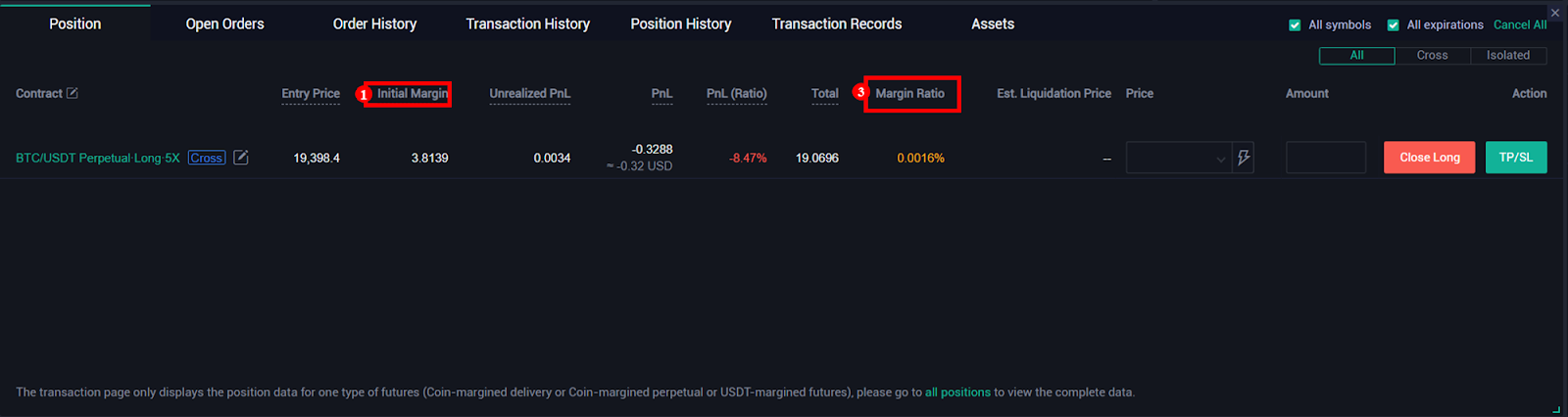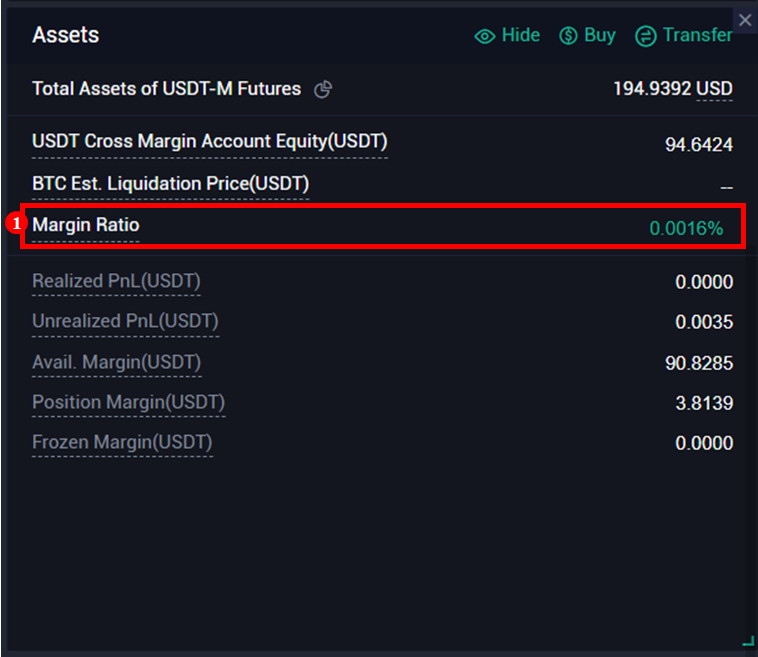# 1.Key Takeaways

Huobi will adjust and upgrade the naming of a few liquidation-related indicators in futures trading. The key change is the adoption of the commonly used term "maintenance margin" in calculating the liquidation indicator.

##1) Replace the field "Position Margin" with "Initial Margin";

2) Fields of Available and Avg. Price are deleted;

3) Take Profit and Stop Loss are merged into TP/SL;

4) Add term "Margin ratio", which is calculated as below:

Margin ratio (Isolated) = Maintenance margin ratio * Leverage for opening the position * Initial margin (Position margin) *100% / Isolated position equity

Margin ratio (Cross) = Maintenance margin ratio * Leverage for opening the positions * ∑All cross margin futures (Used margin) * 100% /(Account equity - ∑All isolated margin futures (Used margin)

(Margin ratio = Maintenance Margin * 100% / Position Equity; Your position will be liquidated)

## （2）Huobi website

1）Positions Zone•  Replace the field "Position Margin" with "Initial Margin";
•  Delete fields: Coverage Ratio, Position Price, and Available;
• Add field "Margin ratio", which is calculated as below:

Margin ratio (Isolated) = Maintenance margin ratio * Leverage for opening the position * Position margin asset *100% / Isolated position equity

Margin ratio (Cross) = ∑(Maintenance margin ratio * Leverage for opening the position * Used margin assets by all cross margin futures ) * 100% /(Cross account equity - ∑In-orders frozen margin assets of all isolated margin futures )

(Margin ratio = Maintenance Margin * 100% / Position Equity; Your position will be liquidated)

2）Assets Zone• Delete fields: Coverage Ratio and Adjustment Factor
•  Add fields: Maintenance Margin and Margin Ratio

Maintenance margin (Isolated) = Maintenance margin ratio * Leverage for opening the position * Position margin assets

Maintenance margin (Cross ) = ∑Maintenance margin ratio * Leverage for opening the position * Used margin assets by all cross margin futures

Margin ratio (Isolated) = Maintenance margin ratio * Leverage for opening the position * Position margin asset *100% / Isolated position equity
Margin ratio (Cross) = ∑(Maintenance margin ratio * Leverage for opening the position * Used margin assets by all cross margin futures ) * 100% /(Cross account equity - ∑In-orders frozen margin assets of all isolated margin futures )

(Margin ratio = Maintenance Margin * 100% / Position Equity; Your position will be liquidated)

Trade on the go with Huobi App (iOS/Android)

Find us on

Telegram:

https://t.me/huobiglobalofficial

https://t.me/HuobiGlobal_zw

https://t.me/HuobiFutures_en

Discord：

https://discord.gg/huobi

Huobi reserves the right in its sole discretion to amend or change or cancel this announcement at any time and for any reasons without prior notice. The above is for information purposes only and Huobi makes no recommendations or guarantees in respect of any virtual asset, product, or promotion on Huobi. Prices of virtual assets are highly volatile and trading virtual assets involves risk. Please read our Risk Reminder text here.